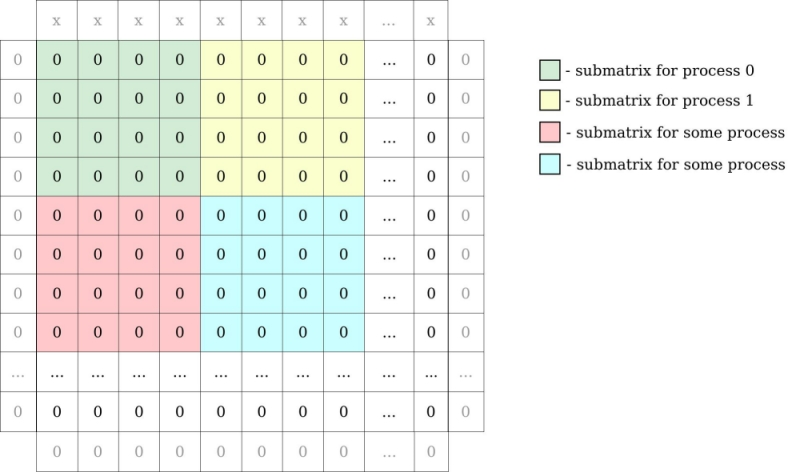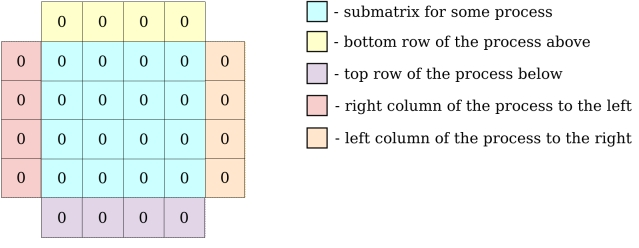# PDI 1.1.0

PDI examples

The example is made of a single source file (one version in each supported programming language), this file loads its configuration and PDI specification tree from a YAML file specified on the command line. Just by replacing the configuration file, the example program will use different PDI plugins, different libraries and different I/O operations.

The example implements implements a simple Heat equation solver using an explicit forward finite difference scheme parallelized with MPI in 2 dimensions.

# Code algorithm

The data is stored in a 2D array in which each point represents the temperature. In every iteration, the value at the next iteration of each cell is computed by applying a cross stencil using the values of the cell and of its neighbours (top, bottom, left and right) at the current iteration:

data[i][j] = 0.500 * data[i][j]
+ 0.125 * data[i-1][j]
+ 0.125 * data[i+1][j]
+ 0.125 * data[i][j-1]
+ 0.125 * data[i][j+1];

In order not to override the cells while processing we use two arrays to store the values, one for the current iteration (`cur` in the code) and one for the next iteration (`next` in the code). These computations are written in the `iter` function.

This code is parallelized with MPI . Let's split that matrix by MPI processes. Each process will compute part of global matrix.

For example: matrix 16 x 16 integers and 16 MPI processes gives submatrices of 4 x 4 integers for every process. We have to add to our global matrix one row above and below, column to the left and right to be able to compute border cells. In our example row on the top has some value (”x”) bigger than 0 (representing source of heat):MPI processes need to exchange information about their local matrix border cells (communicate with neighbours to exchange row/column of matrix). Each MPI process will have a local matrix:All the communications instructions are written in `exchange` function.

# PDI integration

Now, when we know the algorithm, we can focus on analysing `decl_hdf5.yaml` specification tree. Fisrt 3 maps defined will not be seen to PDI:

duration: 0.75
datasize: [60, 12]
parallelism: { height: 3, width: 1 }
• `duration` is the value in seconds how long the application will run.
• `datasize` is size of our global matrix.
• `parallelism` defines the number of MPI processes in each dimension.

Next, we have defined `data` and `metadata`:

pdi:
iter: int
dsize: { size: 2, type: array, subtype: int }
psize: { size: 2, type: array, subtype: int }
pcoord: { size: 2, type: array, subtype: int }
data:
main_field: { size: [ '\$dsize', '\$dsize' ], type: array, subtype: double }

In source file we will extract the `pdi` map and pass it as PDI_init argument.

• `iter` will hold the current iteration number.
• `dsize` will hold the size of local matrix of each MPI process.
• `psize` will hold number of processes in dimensions.
• `pcoord` will hold coordinates for each process.
• `main_filed` is the local matrix for each process.

Let's take a closer look at C source code.

PDI_init(PC_get(conf, ".pdi"));

As mentioned before, we extract the `pdi` subtree and pass it to PDI_init.

We did not defined `mpi_comm` data in yaml, so this line will have no effect:

PDI_expose("mpi_comm", &main_comm, PDI_INOUT);

The same goes for all PDI calls with data we didn't defined.

int dsize;
PC_int(PC_get(conf, ".datasize"), &longval); dsize = longval;
PC_int(PC_get(conf, ".datasize"), &longval); dsize = longval;

Here we are reading global matrix size from specification tree. Similar with parallelism and duration.

After calculating the local matrix sizes and coordinates, we expose them:

PDI_expose("dsize", dsize, PDI_OUT);
PDI_expose("psize", psize, PDI_OUT);
PDI_expose("pcoord", pcoord, PDI_OUT);

At the beginning of each iteration, we call multiexpose:

PDI_multi_expose("newiter",
"iter", &ii, PDI_INOUT,
"main_field", cur, PDI_INOUT,
NULL);

Above instruction will share `iter` and `main_field`, call `newiter` event and then reclaim `main_field` and `iter`. This is the place when plugins will read/write our data.

We have covered the logic behind the PDI example. Now you can start the Hands-on tutorial.